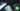# Hackerrank | Solution of Staircase in Golang

September 1st, 2018
|

In this post we will solve question staircase from Hackerrank. A simple one, only requires to decode the logic for pattern. Will need if-else and a nested for loop. Let's get started.

# Problem Statement

The question can be found at this link. The problem statement basically states that we need to print the given pattern for any given number as input. Couple of things to note here is

• We need to take a single integer as input.
• Print the given pattern.
• we are solving this in golang.

# Challenges

• Choose our preferred language as golang on hackerrank. The moment we do that, we get some 50-60 lines of code which are very unfamiliar to someone who is new to language.
• To solve this problem only thing we need to decode the logic for the pattern.
• Formatted printing required.

# Solution

This is the template you get on hackerrank for this problem statement.

``````    package main

import (
"bufio"
"fmt"
"io"
"os"
"strconv"
"strings"
)

// Complete the staircase function below.
func staircase(n int32) {

}

func main() {

checkError(err)
n := int32(nTemp)

staircase(n)
}

if err == io.EOF {
return ""
}

return strings.TrimRight(string(str), "\r\n")
}

func checkError(err error) {
if err != nil {
panic(err)
}
}``````
• First go ahead and delete everything in the pre-existing template.
• Let's write down our main wrapper function.
``````package main
import "fmt"

func main(){

}``````

Next we need to take an input, so let's go ahead declare a variable and take an input for our program.

``````    ...
var n int
fmt.Scan(&n)``````

Now let's look at the pattern and try to uunderstand the logic required to print it.

``````    for an input 4
#
##
###
####
``````

Now let's start noting down the points that would help us in printing this pattern

• The pattern has 4 rows, i.e. equal to input number. So we would need a loop which loops over input times
• It has four columns in every row. We would need inner nested loop, which would also loop over input times
• There are two types of output, either an space or an #. So we need two inner loops instead of one.
• In the first line spaces are 3, # is 1, in second line spaces are 2 and # are 2. If we notice the index of outer loop for the first and second case it is 0,1. So we need to print # `i+i` times. For the spaces, we need to print it `input-index-1` times.

Now that we have figured out the logic of the pattern, let's see how the code goes.

``````    ...
for i:=0;i<n;i++{
for j:=0;j<n-i-1;j++{
fmt.Print(" ")
}
for j:=0;j<=i;j++{
fmt.Print("#")
}
fmt.Println()
}``````

So we have a outer loop which runs `n` times. This to make sure our pattern has `n` rows. Next we have two inner loops, one to print spaces, other for the hash. The first one is running `n-i-i` times and second one `i+1` times. Notice the use of `Print` instead of `Println` , because we need everything to print in one line. Next after the inner loops we print a new line, to move the cursor to next line. This completes our program, and your complete code should look like

``````package main

import "fmt"

func main(){
var n int
fmt.Scan(&n)

for i:=0;i<n;i++{
for j:=0;j<n-i-1;j++{
fmt.Print(" ")
}
for j:=0;j<=i;j++{
fmt.Print("#")
}
fmt.Println()
}
}``````

So this is it for this one guys, complete source code for this one can be found on my Github Repo. Will see you in the next one.